Latest Banking jobs   »   Reasoning Ability Quiz For SBI Clerk...

# Reasoning Ability Quiz For SBI Clerk Prelims 2022- 30th April

Directions (1–5): Following questions are based on the five three-digit numbers given below:

369   717   922   625   434

Q1. If all the numbers are arranged in ascending order from left to right, which of the following will be the product of the first and last digits of the number which is in the middle in the new arrangements?
(a) 16
(b) 30
(c) 27
(d) 49
(e) 18

Q2. If the positions of the first and third digits of each of the number interchange, what will be the sum of all the digits of the second lowest number?
(a) 13
(b) 15
(c) 16
(d) 11
(e) 20

Q3. If one is subtracted from each odd digit and two is added to each even digit of each of the numbers, what will be the difference between the third digit and the first digit of the lowest number?
(a) Zero
(b) 6
(c) 4
(d) 2
(e) 5

Q4. What will be the resultant if the first digit of the highest number is divided by the third digit of the lowest number?
(a) 0.5
(b) 1
(c) 2
(d) 2.2
(e) None of these

Q5. If all the digits in each of the numbers are arranged in descending order within the number from left to right, which of the following will be the second highest number in the new arrangement
(a) 369
(b) 717
(c) 922
(d) 625
(e) None of these

Directions (6-7): Study the following information carefully and answer the question given below-
There are eight members in a family i.e., K, O, L, N, Q, M, P and S. Among them three are married couples. S is the daughter of the one who is L’s nephew. Q is the daughter-in-law of the one who is grandfather of M. O has only one grandson. P is grand-daughter-in-law of K. L is O’s husband’s sister.

Q6. How many children P’s father-in-law has?
(a) Two
(b) One
(c) Three
(d) Four
(e) None of these

Q7. Who among the following is father of N?
(a) O
(b) L
(c) K
(d) M
(e) None of these

Directions (8-12): Study the following information carefully and answer the question given below:
M, N, O, P, Q, R, J and K are sitting in a straight line but not necessarily in the same order. Some of them are facing south while some are facing north. Only two people sit to the right of M. N sits third to the left of M. Only one person sits between N and R. R sits to the immediate right of Q. Only one person sits between Q and K. Both the immediate neighbours of N face the same direction. M faces north. O sits third to the left of R. N faces the opposite direction of M. J does not sit at any of the extremes ends of the line. P faces the same direction as Q. Both J and O face the opposite direction of K.

Q8. How many persons in the given arrangement are facing North?
(a) More than four
(b) Four
(c) One
(d) Three
(e) Two

Q9. Four of the following five are alike in a certain way, and so form a group. Which of the following does not belong to the group?
(a) Q, R
(b) K, J
(c) N, M
(d) N, J
(e) P, O

Q10. What is the position of R with respect to K?
(a) Second to the left
(b) Third to the right
(c) Third to the left
(d) Fifth to the right
(e) Second to the right

Q11. Who amongst the following sits exactly between K and Q?
(a) N
(b) J
(c) R
(d) Q
(e) O

Q12. Who is sitting 2nd to the right of N?
(a) K
(b) P
(c) R
(d) Q
(e) None of these.

Directions (13-15): Study the following information carefully and answer the questions given below:
Point A is 6m west of point B, which is 8m south of point F. Point D is 26m east of point C. Point G is 15m west of point H. Point C is 5m north of point A. Point H is 17m north east of point F. Point D is 10m south of point E.

Q13. What is the shortest distance between point G and point F?
(a) 16m
(b) 10m
(c) √5m
(d) 8m
(e) None of these

Q14. What is the direction of point E with respect to point B?
(a) North-east
(b) South
(c) North
(d) West
(e) None of these

Q15. What is the shortest distance and direction of point G with respect to point B?
(a) 18m, South-west
(b) 15m, South
(c) 16m, North
(d) 20, North-west
(e) None of these

Solutions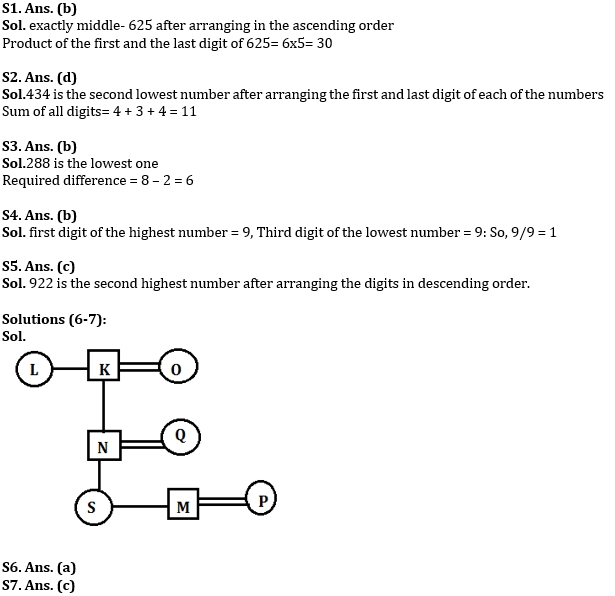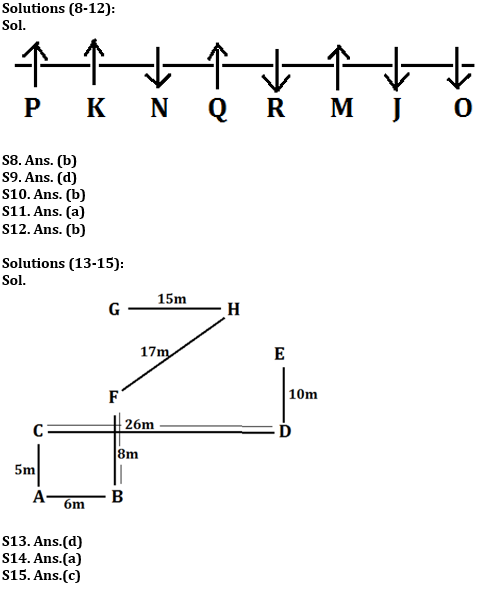#### Congratulations!

Incorrect details? Fill the form again here

•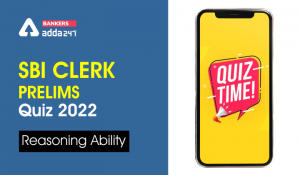Reasoning Ability Quiz For SBI Clerk Pre...
•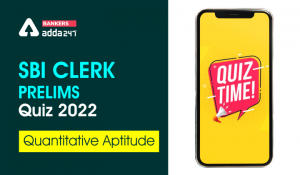Quantitative Aptitude Quiz For SBI Clerk...
•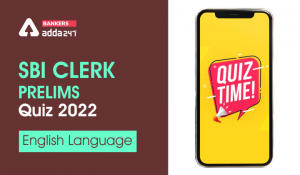English Quizzes For SBI Clerk Prelims 20...
•Reasoning Ability Quiz For SBI Clerk Pre...
•English Quizzes For SBI Clerk Prelims 20...
•Reasoning Ability Quiz For SBI Clerk Pre...